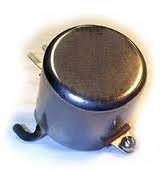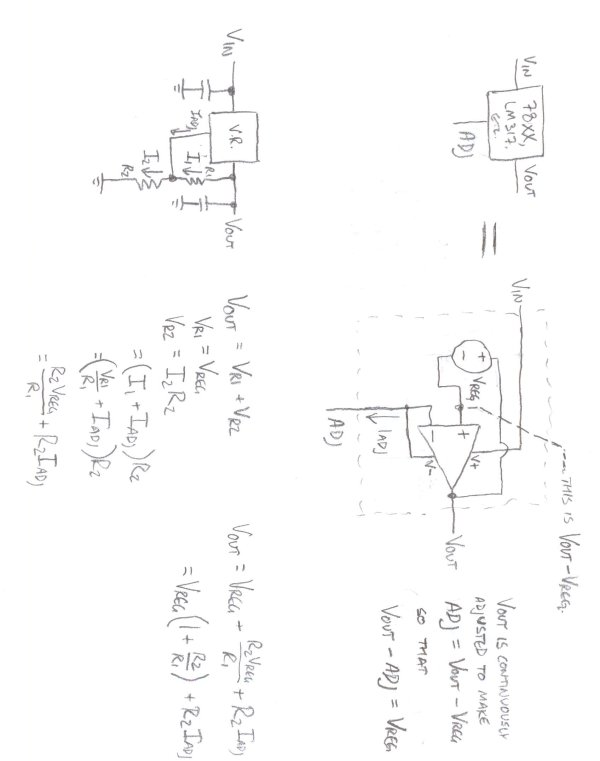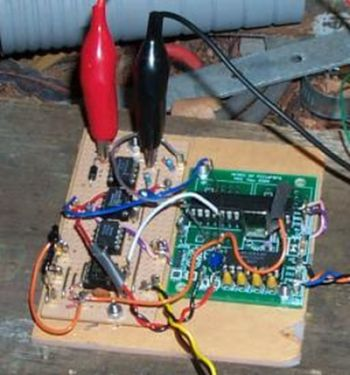# Fun with Voltage Regulators

I’ve been spending a lot of time thinking about op amps and comparators lately.

One of the common uses of an op amp is as a “unity gain” buffer, meaning that the output voltage is as close as possible to being the same as the input voltage. At a glance this might seem like a trivial thing, but it’s used to great advantage.

For example, a resistive voltage divider can be used to derive some fraction of a larger voltage by representing the ratio with resistors. Given a 9V supply, a divider over 100K and 47K would yield about 2.9V (9V * 47K/(100K+47K)). Unfortunately, this is of only limited use directly, since driving a load from the output causes that voltage to drop. This happens because the load itself acts as a resistor to ground in parallel with the divider, changing its resistance.The divider does okay, though, feeding to a high-impedance input (i.e., one that doesn’t pull a lot of current). The inputs on an op amp fit that description, and its output is low-impedance (meaning that it can supply current without trouble). That’s a huge part of why op amps are useful. If you take the resistive divider’s output into the non-inverting (“+”) input of an op amp and the amp’s own output into the inverting (“-“) input, you get a unity gain buffer, an amplifier that outputs the same amplitude as the input (“unity gain” refers to the 1:1 ratio of input to output). The buffer continuously compares its output to the input. If the output is too high, it’s lowered; if too low, it’s raised.

READ  Christmas card with inbuilt retro video game for under \$10

In general, the op amp’s job is to perform an analog computation on its inputs that amounts to subtraction followed by multiplication. It subtracts the voltage of the inverting input from the voltage of the non-inverting input, then multiplies it by a factor called gain. By default, the gain is an arbitrarily high number called the open-loop gain of the amp. However, by feeding the output back into the inputs in different ways, it’s possible to fix the gain to a more useful value, such as 1 in the above scenario.

Anyway, this ability to continuously adjust an output to a certain point reminded me of linear voltage regulators, like the 7805 and the LM317. I hypothesized that these 3-terminal regulators are basically heavy-duty op amps with a fixed voltage reference on one of the inputs. A little research confirmed this.

[Note: The above image replaces a previous version that was less accurate.]

The data sheet for the fixed 7805 (and other 78xx) regulators suggested a circuit that allows the use of the regulator in an adjustable mode for voltages higher than the nominal voltage. Under normal circumstances, the regulator simply adjusts the output pin to be exactly 5V above the ground pin. In this mode, the ground pin is not tied to ground. Instead, the output and ground pin are placed across the high side of a resistive divider. The regulator fixes the voltage across the upper resistor to its face value. The lower resistor gets all of the current from the upper resistor, plus the garbage quiescent current from the machinery in the device. Multiplying this sum by the lower resistance yields, via Ohm’s law, the voltage across the lower resistor. The sum of that voltage and the regulated voltage is the output.

The data sheet for the LM317 shows a circuit essentially identical to the one described above with a few name changes. In fact, the ‘317, when used in the 7805’s nominal configuration, is itself a fixed 1.25V regulator.Knowing that these regulators are so alike, then, why should we ever choose ‘317 over 7805? The answer: ‘317 has a subtle difference that makes it far more suitable in its adjustable mode: The current out of its adjustment terminal is a couple of orders of magnitude smaller enough smaller in fact that it could be disregarded in many cases when calculating Vout. This is especially useful in cases where the application doesn’t adjust the output voltage, but that requires a regulator for an awkward voltage (like the 13V programming voltage of a PIC). Using a 7805 in such an application might require a trimmer to fine-tune the output, while the ‘317 would probably be okay with a fixed-value resistor instead.

Pictured are the much simplified equivalent schematics, plus my derivation of how Vout is calculated. R2Iadj should be dominated by the rest of the expression.

For more detail: Fun with Voltage Regulators